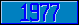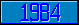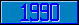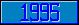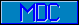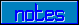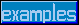# Operators

Arithmetic operator plus (+)
Arithmetic operator minus (-)
Arithmetic operator times (*)
Arithmetic operator divided by (/)
Arithmetic operator integer divided by (\)
Arithmetic operator modulo (#)
Arithmetic operator to the power of (**)
String operator concatenate (_)
Assignment operator becomes (=)
Relational operator is equal to (=)
Relational operator object is equal to (==)
Relational operatr is greater than (>)
Relational operator is less than (<)
Relational operator is less than or equal to ('>)
Relational operator is greater than or equal to ('<)
Relational operator matches pattern (?)
Relational operator contains ([)
Relational operator follows (])
Relational operator follows or is equal to (]=)
Relational operator collates after (]])
Relational operator collates after or is equal to (]]=)
Logical operator and (&)
Logical operator or (!)
Locical operator exclusive or (!!)
Logical unary operator not (')
Indirection operator indirect (@)

The M[UMPS] programming language knows a number of 'operators'. Some operators are the familiar arithmetic ones while others operate on strings or compare values. In M[UMPS], the operators can be mixed in any order, but keep in mind that some operators imply a specific interpretation of their operands. The only order of precedence for binary operators in M[UMPS] is the strict left- to-right evaluation, and unary operators only apply in a right- to-left order. If that order of precedence needs to be overridden, parentheses can be used to indicate that a sub- expression has to be evaluated first.

Depending on where one went to school, different schemes for the order of computations were taught. The main different 'schools' that I am aware of are:

Central Europe   USA and Great Britain
1.Raising to a power1.Raising to a power (including taking roots)
2.Multiplication
3.Division
4.Take root2.Multiplication or division

So that the result of: 4/2*2 is equal to 1 in The Netherlands (multiplication takes precedence over division), but equal to 4 in England (multiplication and division have no difference in precedence, so the left-to-right evaluation prevails).

M[UMPS] evaluates strictly from left to right, so that 1+1*2 yields 4 and not 3.

When a different precedence is to be established, parentheses should be used: the expressions 1+(1*2) and 1*2+1 will both yield 3.

Assume that K=34; the expression "_11_22_33_"["_"_K_"_" will evaluate as "134_". The order of evaluation is: first evaluate "_11_22_33_"["_", which yields 1 (true), then the rest of the expression becomes: 1_K_"_", which leads to the value "134_".
If one wished to perform a check to verify that the value of K is contained in a list, parentheses are required:
"_11_22_33_"[("_"_K_"_") will return a true-or-false value (bve, boolean valued expression), which is only true when K has one of the values 11, 22 or 33.

Assume that L=29 and K=34; the series of commands
If K=L+3 Write !,"K=32"
will result in the text "K=32" being printed, regardless of the value of K.
In this case, the order of evaluation is: first compare K=L, which will yield 0 (false), then the evaluation continues with 0+3, which yields 3 (true), so that the commands following the If command will always be processed.
The formula that was intended could be either L+3=K or K=(L+3).